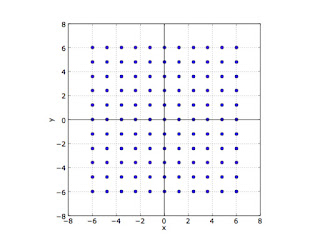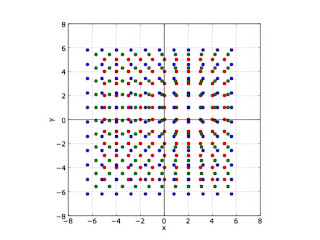## Sunday, March 11, 2012

### Geocentrism & Cosmological Redshift

Laurence Krauss had a simple demonstration (see Reading: A Universe from Nothing by Lawrence Krauss) of how expansions where the recessional velocity is proportional to the distance of the object create a system where a center cannot be defined from inside the distribution.  This is the same type of expansion revealed in extra-galactic redshifts.

This type of expansion is more generally called homologous expansion.  Ned Wright as some graphics describing various types of expansions on his cosmology site: "Distortion during Expansion of the Universe"

Stepping Through the Expansion

Paralleling the treatment in Krauss, we'll first take a few snapshots of the positions of our galaxies at three different times.  The positions of the galaxies, where our observers are located, are called the co-moving frame, and are carried with the expansion.  At the first time, all the points (galaxies) are located at the vertices of unit squares, so we have galaxies at (x,y)= (0,0), (1,0), (1,1), etc. which are represented by the red dots in this graphic.  At each time step we expand the distance between each of the points.Figure 1: scale = 1.0

At the next timestep, the distances between each of the points is expanded by a factor of 1.2.  The co-moving coordinates of each galaxy remain the same, but the measured distances between the galaxies has increased.Figure 2: scale = 1.2

For good measure, we'll expand the scale once more by a factor of 1.4 over the original scale.  Assuming the same time between each step, this corresponds to a constant rate of expansion.Figure 3: scale = 1.4

To explore this exercise yourself, print out the three graphs above on thin paper (sufficiently translucent that you can read other material trough it, or are able to hold it up to a light).

Now we overlay the three plots, lining up the dots at (0,0), representing our home galaxy, for each plot.  If you've printed these out on sufficiently translucent paper, you should be able to line up the three pages (or at least two of the pages) and see through them to see the overall picture.  When properly aligned, it would look something like Figure 4:Figure 4: Overlay at (0,0)

Here we see an analog of the cosmological motions with how the dots overlap, corresponding to later times.  The galaxy at (0,0) stays fixed, the (apparent) center of the motion.  But as we look at objects away from the center, the dots marking the galaxies nearest the center are still so close, the dots corresponding to different times slightly overlap - their velocities are low.  But further out, the distances between the corresponding galaxies (red-to-green-to-blue) get further apart for the same amount of time.  Therefore the galaxies further away from the center appear to be moving faster.   The greater separation will be in proportion to the distance of the object from the center, analogous to the Hubble law.

But suppose we want to see how things look from one of the other galaxies?

Let's adjust the plot so we observe the expansion from the galaxy at appears to start at the position (2,1) in the first graphic.  We can do the same with our printed pages.  Be careful to align the SAME co-moving galaxy of the observer on each page.  So we overlay the corresponding point in the remaining plots and getFigure 5: Overlay at (2,1)

Here we see the exact same thing as before.  The galaxy at (2,1) corresponding to our observer remains motionless in this frame, while as we move further out, we see the galaxies appear to be organized concentrically around this new 'center', with low velocities near the observer galaxies and higher velocities further away.

This analogy also works in 3-dimensions.

This is why we can say the Hubble expansion cannot define a center.  To any observer, located anywhere, they will perceive themselves the center of the expansion.

We're still in the Center of the Circle!
One of the popular responses to the fact demonstrated above is how we appear to be in the center of galaxy catalogs such as the SDSS survey.  Note that I could have expanded the assumption of uniformity above by expanding the plot of points (galaxies) such that the edge was not visible - and the conclusions would not be altered.

Delusions of Geocentric Quantization...

However, as I demonstrated in some posts in my quantized redshifts series, a uniform distribution in space, which is magnitude limited (i.e. there are galaxies fainter than can be detected by our instruments) will exhibit a similar distribution with the galaxies which we can detect. For details, see:
That the surveys do have a magnitude limitation is reinforced by the second 'hump' in the SDSS N-z curve.  That extra hump is due to more galaxies discovered with instruments that could see to fainter magnitudes, suggesting that there are substantially more galaxies which we might see with more sensitive instruments.

That this works provides a consistency check against our other assumptions, such as the redshifts being an actual measure of distance and not intrinsic properties of the galaxies.

johnmartin said...

What is the probability of ten bodies with random motion, moving apart along the same line of motion as required in a 2D uniformly expanding universe of 100 bodies?

Is the probability so small that we should not even consider the expanding universe seriously. If not why not?

JM

W.T."Tom" Bridgman said...

To John Martin,

Thinking of the Big Bang as an explosion is a common misconception. If I attach those bodies on a rubber sheet, and then stretch the rubber sheet, they will all expand from each other in a fashion similar to the Big Bang.

The balloon analogy is even better.
YouTube: Expansion of the Universe: Where is the Centre? - The Balloon Analogy

AstronomyCafe: The Big Bang was NOT a Fireworks Display!

Angry Astronomer: The Big Bang – Common Misconceptions

American Institute of Physics: Big Bang or Steady State?

Harvard CFA: Brief Answers to Cosmic Questions: Did the Universe expand from a point? If so, doesn't the universe have to have an edge?

Wikipedia: Big Bang

LiveScience: Was the Big Bang Really an Explosion?

john martin said...

Tom

There is a problem with the lattice model. Either the forces that cause the universe to expand are random, or controlled by design. If random, then the uniform motion of the galaxies relative to each other comes under a probability analysis, just like tossing a coin to get either heads or tails.

Once we line up a 10 by 10 2D lattice and claim they are all uniformly moving apart due to random forces, then the probability that such a universal, uniform expansion can occur is effectively zero.

If we do a rough calculation and say the chance of an object moving in any direction is 1/360. Then the chance of two objects moving in the exact opposite direction to each other is 1/360 x 1/360. Let Y = 1/360 x 1/360 and then three objects moving uniformly apart in opposite directions is (Y)^3. Likewise 4 objects (Y)^6 and five objects (Y)^10. From this simple analysis, 4 objects moving apart from each other along the same line of motion due to random forces is effectively zero. Therefore the lattice example has a probability of zero of occurring.

If we are very generous and say 0.5 probability of two objects moving relative to each other along the same line. Then 5 objects have (0.25)^10=9.54x10^-5% chance of occurring. Such a probability is effectively zero for only five objects moving uniformly in one direction. If we have 5 objects aligned vertically and 5 objects aligned horizontally in a symmetric cross, then the probability of all these objects expanding uniformly along the x and y axes is not more than 9.09x10^-9% of occurring. Evidently the lattice example is a maths fiction.

If however the big bang occurred due to controlled forces by design, then the lattice example may occur. But modern science has not proposed any satisfactory, physical model to account for such forces occurring in a controlled way.

Therefore the lattice example is special pleading by

1) ignoring the zero probability of a lattice structure uniformly expanding in all directions

2) Ignoring the necessity of having the model proposed outside of a known science mechanism that produces a controlled force structure.

The problem for the uniformly expanding lattice is far worse than proposed here, for

1) The lattice must expand uniformly in all directions and not just along one line of motion as proposed in the example above.

2) The objects are not moving uniformly apart in the BB model, but the space between the objects is expanding uniformly between all objects. Yet there is no solid empirical science experiments that have been performed to show physical space really does or can expand. Therefore the BB model assumes an unscientific, non-experiment based assumption concerning the nature of space and expansion. Therefore the BB theory is not scientific and the lattice model is an unscientific maths model that requires special pleading concerning the nature of a maths model that is said to have explanatory power apart from science experiment.

Unless solid answers are proposed to counter this simple invalidation of the lattice model, the big bang principle of uniform expansion is a mathematical and science myth.

JM

W.T."Tom" Bridgman said...

John,

You're making the same error as before.

The structure doesn't have to be a lattice. I chose a lattice structure just so a uniform scale change is obvious to casual observer. The geometry works just fine for randomly distributed particles, or do you need to see a demonstration?

It is not due to a 'force structure' as due to the underlying changing geometry of space which essentially 'carries' the galaxies along like motion of boats on a river (hence the occasional term 'Hubble flow'). What is the probability of objects floating in a uniformly flowing river being carried together down the river? That this structure expands uniformly is a consequence of the scaling term, a(t), in the FLRW metric which is itself a consequence of geometric isotropy and homogeneity.

As for the reality of the expansion as it has not been experimentally measured locally, the same could be said for gravitation prior to the 1950s. We had NO experimental evidence that the planets moved due to the same force we measured on Earth, as recently as the 1950s. We just had equations that could accurately predict planetary motions. We could not do the "Newton's Cannon" experiment (Wikipedia) until we did space flight (or do you believe space flight is a hoax?). Of course, if the U.S. had used your argument that Newtonian gravity had not been laboratory tested, it would not have been reasonable to pursue space flight. If that were the case, you'd probably be speaking Russian now.

Either way, like gravity before space flight, the observations are consistent with space expanding, and mathematically consistent with the theory of gravity. The expansion does resolve the annoying problems with making an 'explosion' mechanism work, by arguments not that different from what you present.

Unknown said...

Could you summarize what Mr. Martin was on about and why he was wrong?

W.T."Tom" Bridgman said...

To Jacob,

I've posted some newer material on the topic at Geocentrism: Mach, 'Aether Drag' and Aberration.